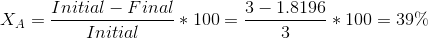# AP Chemistry : Kinetic Molecular Theory

## Example Questions

### Example Question #9 : Thermochemistry And Kinetics

Two moles of nitrogen gas are kept in a glass container. The temperature of the gas is 400K.

What is the kinetic energy of the gas?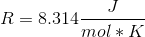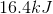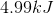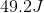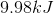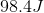Explanation:

Using the equation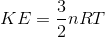, n being the number of moles, R being 8.314, and T being the temperature in Kelvin, we can find the kinetic energy of two moles of gas in a container.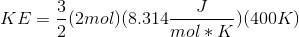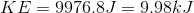### Example Question #1 : Kinetic Molecular Theory

Which of the following is not an assumption of the kinetic molecular theory of gases?

Gas particles do not experience attractions/repulsions to other particles

Collisions between gas particles are elastic

Gas particles are in random, continuous motion

The volumes of the particles of a gas are negligible

The kinetic energy of gas particles are different for all gases at a certain temperature

The kinetic energy of gas particles are different for all gases at a certain temperature

Explanation:

The kinetic molecular theory of gases states that the average kinetic energy of gas particles is proportional to temperature, and it is the same for all gases at a given temperature. This is the opposite of what is stated in the answer choice.

### Example Question #1 : Kinetic Molecular Theory

Why does a higher temperature result in a faster reaction?

It helps orient reactants into the correct position to react

It increases the surface area for reactions

It increases the pressure of the reactants

It increases the number of effective molecular collisions

It lowers the activation energy for a reaction

It increases the number of effective molecular collisions

Explanation:

The temperature of a system can be manipulated to increase the reaction rate. The temperature affects the rate of the reaction because as temperature increases, so does the average kinetic energy. This means that molecules (reactants) are moving around more quickly, resulting in more frequent molecular collisions, resulting in an increased rate of product formation.

### Example Question #2 : Kinetic Molecular Theory

Which of the following statements in not an assumption made in kinetic molecular theory?

Collisions between gas molecules and the container are perfectly elastic

No attractive forces exist between gas molecules

The average kinetic energy of gas molecules is a function of both temperature and pressure

Much of the volume of the container is empty space

The average kinetic energy of gas molecules is a function of both temperature and pressure

Explanation:

The average kinetic energy is considered to be a sole function of temperature; pressure is not a factor. All of the other statements are assumptions of kinetic molecular theory.

### Example Question #3 : Kinetic Molecular Theory

The reaction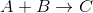obeys the rate law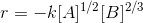What are the units of the rate constant,?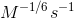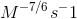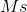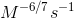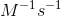Explanation:

Recall that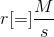and thatandare both molar concentrations. Then,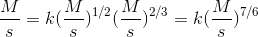Dividing both sides by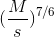gives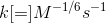### Example Question #4 : Kinetic Molecular Theory

Consider the following first order reaction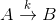Where k is the rate constant.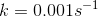and the initial concentrations of A and B are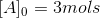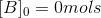What is the percent conversion after 500 seconds? Round to the nearest percent.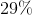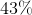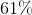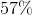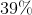Explanation:

For a first order reaction,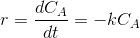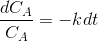Integrating both sides gives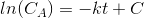Whereis an integration constant.

Solving for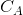gives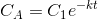Initially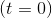there are 3 mols of. Using this we find that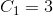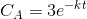At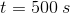,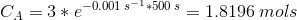Percent conversion is calculated as follows: